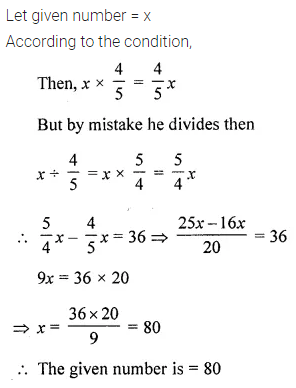# ML Aggarwal Class 8 Solutions for ICSE Maths Chapter 1 Rational Numbers Ex 1.6

## ML Aggarwal Class 8 Solutions for ICSE Maths Chapter 1 Rational Numbers Ex 1.6

Question 1.
In a bag, there are 20 kg of fruits. If 7$$\frac { 1 }{ 6 }$$ kg of these fruits be oranges and 8$$\frac { 2 }{ 3 }$$ kg of thee are apples and rest are grapes. Find the mass of the grapes in the bag.
Solution:Question 2.
The population of a city is 6,63,432. If $$\frac { 1 }{ 2 }$$ of the population are adult males and $$\frac { 1 }{ 3 }$$ of the population are adult females, then find the number of children in the city.
Solution: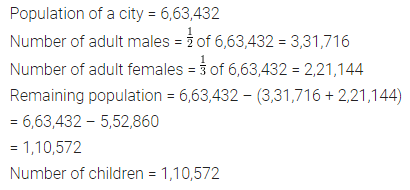Question 3.
In an election of housing society, there are 30 voters. Each of them gives the vote. Three persons X, Y and Z are standing for the post of Secretary. If Mr X got $$\frac { 2 }{ 5 }$$ of the total votes and Mr Z got $$\frac { 1 }{ 3 }$$ of the total votes, then find the number of votes which Mr Y got.
Solution: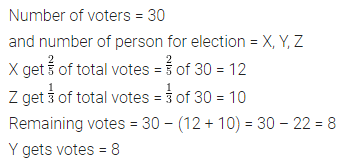Question 4.
A person earns ₹ 100 in a day. If he spent ₹ 14$$\frac { 2 }{ 7 }$$ on food and ₹ 30$$\frac { 2 }{ 3 }$$ on petrol. How much did he save on that day?
Solution:Question 5.
In an examination, 400 students appeared. If $$\frac { 2 }{ 3 }$$ of the boys and all 130 girls passed in the examination, then find how many boys failed in an examination?
Solution:Question 6.
A car is moving at the speed of 40$$\frac { 2 }{ 3 }$$ km/h. Find how much distance will it cover in $$\frac { 9 }{ 10 }$$ hrs?
Solution: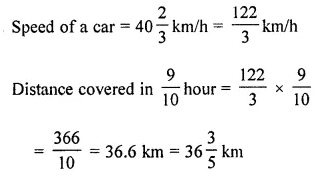Question 7.
Find the area of a square lawn whose one side is 5$$\frac { 7 }{ 9 }$$ m long.
Solution:Question 8.
Perimeter of a rectangle is 15$$\frac { 3 }{ 7 }$$ m. If the length is 4$$\frac { 2 }{ 7 }$$ m, find its breadth.
Solution: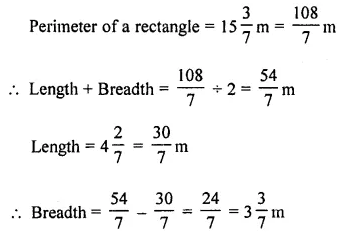Question 9.
Rahul had a rope of 325$$\frac { 4 }{ 5 }$$ m long. He cut off a 150$$\frac { 3 }{ 5 }$$ m long piece, then he divided the rest of the rope into 3 parts of equal length. Find the length of each part.
Solution:Question 10.
If 3$$\frac { 1 }{ 2 }$$ litre of petrol costs ₹ 270$$\frac { 3 }{ 8 }$$, then find the cost of 4 litre of petrol.
Solution:Question 11.
Ramesh earns ₹ 40,000 per month. He spends $$\frac { 3 }{ 8 }$$ of the income on food, $$\frac { 1 }{ 5 }$$ of the remaining on LIC premium and then $$\frac { 1 }{ 2 }$$ of the remaining on other expenses. Find how much money is left with him?
Solution: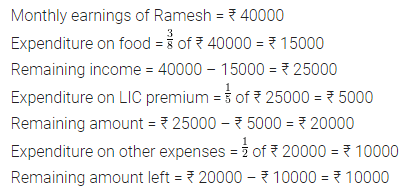Question 12.
A, B, C, D and E went to a restaurant for dinner. A paid $$\frac { 1 }{ 2 }$$ of the bill, B paid $$\frac { 1 }{ 5 }$$ of the bill and rest of the bill was shared equally by C, D and E. What fraction of the bill was paid by each?
Solution:Question 13.
$$\frac { 2 }{ 5 }$$ of total number of students of a school come by car while $$\frac { 1 }{ 4 }$$ of students come by bus to school. All the other students walk to school of which $$\frac { 1 }{ 3 }$$ walk on their own and the rest are escorted by their parents. If 224 students come to school walking on their own, how many students study in the school?
Solution:Question 14.
A mother and her two sons got a room constructed for ₹ 60,000. The elder son contributes $$\frac { 3 }{ 8 }$$ of his mother’s contribution while the younger son contributes $$\frac { 1 }{ 2 }$$ of his mother’s share. How much do the three contribute individually?
Solution:Question 15.
In a class of 56 students, the number of boys is $$\frac { 2 }{ 5 }$$ th of the number of girls. Find the number of boys and girls.
Solution: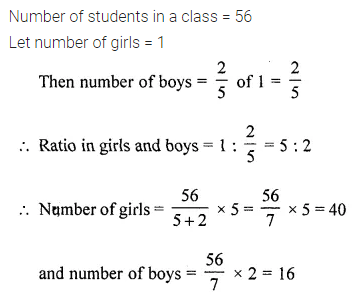Question 16.
A man donated $$\frac { 1 }{ 10 }$$ of his money to a school, $$\frac { 1 }{ 6 }$$ th of the remaining to a church and the remaining money he distributed equally among his three children. If each child gets ₹ 50000, how much money did the man originally have?
Solution: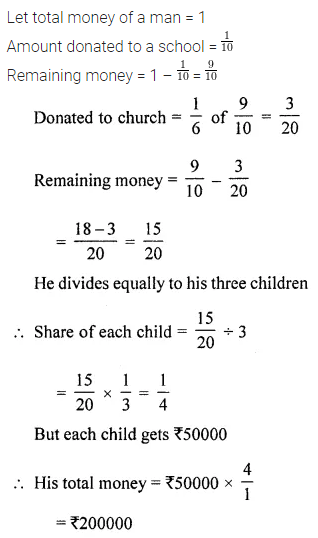Question 17.
If $$\frac { 1 }{ 4 }$$ of a number is added to $$\frac { 1 }{ 3 }$$ of that number, the result is 15 greater than half of that number. Find the number.
Solution: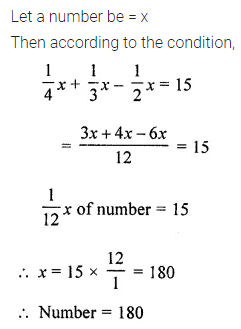Question 18.
A student was asked to multiply a given number by $$\frac { 4 }{ 5 }$$. By mistake, he divided the given number by $$\frac { 4 }{ 5 }$$. His answer was 36 more than the correct answer. What was the given number?
Solution: